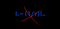# NeoClassical Relativity

## The dynamics of “Space” that generate “Time”…

It is often said that the failure to detect the so-called “Luminiferous Aether” — the postulated medium for the propagation of light — was the first step on the road to Special Relativity. But truth be told, Einstein’s theory made no mention or use of “The Aether”.

Nevertheless, although he did not use…

# Special Relativity Rebooted— Part 3

## The “Aether” Rebooted…

Einstein’s Theory of Special Relativity was undoubtedly a major intellectual breakthrough, but it has been too long burdened with paradoxical nonsense, which only serves to obscure both, the true message of Relativity, and the true nature of the underlying physics of “Time”…

It is often said that the failure to detect the so-called “Luminiferous Aether” — the postulated medium for the propagation of light — was the first step on the road to Special Relativity. But in truth, Einstein’s theory made…

# Absolute Motion

## Is it really a redundant concept?

Maxwell’s Electromagnetic Wave Equation is traditionally read as a three-dimensional wave equation containing a fixed speed of light. An alternative way to read the mathematics is that

NeoClassical Relativity (NCR) is based on this interpretation of Maxwell’s mathematics.

In previous posts, I introduced the two postulates of NeoClassical Relativity.

The first postulate is that: The Speed of Light is a three-dimensional combination of the Speed of Oscillation and Speed of Linear Travel. Mathematically, this postulate can be written as the “3D Speed Equation”

Equation (1) __________ c² = u² + v²

# Length Contraction

## Is it really a “real” thing?Crazy Nonsense — But required by a “fixed” speed of light…

Maxwell’s Electromagnetic Wave Equation is traditionally read as a three-dimensional wave equation containing a fixed speed of light. An alternative way to read the mathematics is that

NeoClassical Relativity (NCR) is based on this interpretation of Maxwell’s mathematics.

My reformulation of Special Relativity is based on the premise that The Speed of Light is a three-dimensional combination of the Speed of Oscillation and Speed of Linear Travel. Mathematically, this postulate can be written as the “3D Speed Equation”

Equation (1) ________________ c² = u² + v²

{Where, (u) is the speed…

# Special Relativity Rebooted — Part 2

## Spacetime Rebooted…

Maxwell’s Electromagnetic Wave Equation is traditionally read as a three-dimensional wave equation containing a fixed speed of light. An alternative way to read the mathematics is that

NeoClassical Relativity (NCR) is based on this interpretation of Maxwell’s mathematics.

In previous posts, I introduced the two postulates of Neo-Classical Relativity.

The first postulate is that: The Speed of Light is a three-dimensional combination of the Speed of Oscillation and Speed of Linear Travel. Mathematically, this postulate can be written as the “3D Speed Equation”

Equation (1) _______________ c² = u² + v²

# Speed of The Cycle — Part 2

## Why differences in the speed of light are hard to detect…

Maxwell’s Electromagnetic Wave Equation is traditionally read as a three-dimensional wave equation containing a fixed speed of light. An alternative way to read the mathematics is that

NeoClassical Relativity (NCR) is based on this interpretation of Maxwell’s mathematics.

In previous posts, I introduced the two postulates of NeoClassical Relativity (NCR).

My first postulate is that: The Speed of Light is a three-dimensional combination of the Speed of Oscillation and Speed of Linear Travel. Mathematically, this postulate can be written as the “3D Speed Equation”

Equation (1) _____________ c² = u² +…

# Special Relativity Rebooted — Part 1

Maxwell’s Electromagnetic Wave Equation is traditionally read as a three-dimensional wave equation containing a fixed speed of light. An alternative way to read the mathematics is that

NeoClassical Relativity (NCR) is based on this interpretation of Maxwell’s mathematics. ©

In previous posts, I introduced the two postulates of NeoClassical Relativity.

The first postulate is that: The Speed of Light is a three-dimensional combination of the Speed of Oscillation and Speed of Linear Travel. Mathematically, this postulate can be written as the “3D Speed Equation”

Equation (1) ______________ c² = u² +…

# Limits of The Cycle

## From minimum existence to maximum existence…

Maxwell’s Electromagnetic Wave Equation is traditionally read as a three-dimensional wave equation containing a fixed speed of light. An alternative way to read the mathematics is that

NeoClassical Relativity (NCR) is based on this interpretation of Maxwell’s mathematics.

In previous posts, I introduced the two postulates of NeoClassical Relativity.

The first postulate is that: The Speed of Light is a three-dimensional combination of the Speed of Oscillation and Speed of Linear Travel. Mathematically, this postulate can be written as the “3D Speed Equation”

Equation (1) ________________ c² = u² + v²

# Duration of The Cycle

## Time Dilation on the Scale of a Single Cycle…

Maxwell’s Electromagnetic Wave Equation is traditionally read as a three-dimensional wave equation containing a fixed speed of light. An alternative way to read the mathematics is that

NeoClassical Relativity (NCR) is based on this interpretation of Maxwell’s mathematics.

In the two previous posts, I introduced the two postulates of NeoClassical Relativity.

The first postulate is that: The Speed of Light is a three-dimensional combination of the Speed of Oscillation and Speed of Linear Travel. Mathematically, this postulate can be written as the “3D Speed Equation”

Equation (1) _________________ c² = u²…

# Length of The Cycle

## All observers agree they see is a Cycle; what they disagree on is the Cycle-Length…

Maxwell’s Electromagnetic Wave Equation is traditionally read as a three-dimensional wave equation containing a fixed speed of light. An alternative way to read the mathematics is that

NeoClassical Relativity (NCR) is based on this interpretation of Maxwell’s mathematics.

In the previous post, I introduced the first of two postulates of NeoClassical Relativity.

The first postulate is that:

The Speed of Light is a three dimensional combination of the Speed of Oscillation and the Speed of Linear Travel.

Mathematically, this postulate can be written as the “3D Speed Equation”

Equation (1):_______________ c²…